TitleCollege Algebra
Answer/Discussion to Practice Problems
Tutorial 6: PolynomialsAnswer/Discussion to 1a -10

 Since the degree of the polynomial is the highest degree of all the terms, it looks like the degree is 0.  Since there is one term, this is a monomial.Answer/Discussion to 1b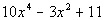Since the degree of the polynomial is the highest degree of all the terms, it looks like the degree is 4.  Since there are three terms, this is a trinomial.Answer/Discussion to 1c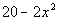Since the degree of the polynomial is the highest degree of all the terms, it looks like the degree is 2.  Make sure that you don't fall into the trap of thinking it is always the degree of the first term.  This polynomial is not written in standard form (descending order).  So we had to actually go to the second term to get the highest degree.   Since there are two terms, this is a binomial.Answer/Discussion to 2a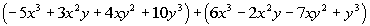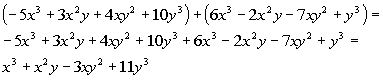*Remove the (  ) *Add like terms togetherAnswer/Discussion to 2b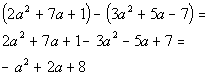*Dist. the - through second ( ) *Add like terms togetherAnswer/Discussion to 2c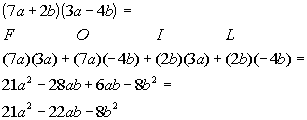*Use the FOIL method *Combine like termsAnswer/Discussion to 2d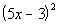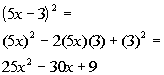*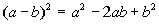Answer/Discussion to 2e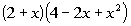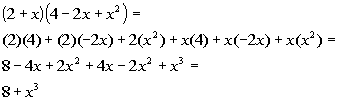*Use Dist. Prop. twice *Combine like terms

Last revised on Dec. 21, 2009 by Kim Seward.
All contents copyright (C) 2002 - 2010, WTAMU and Kim Seward. All rights reserved.